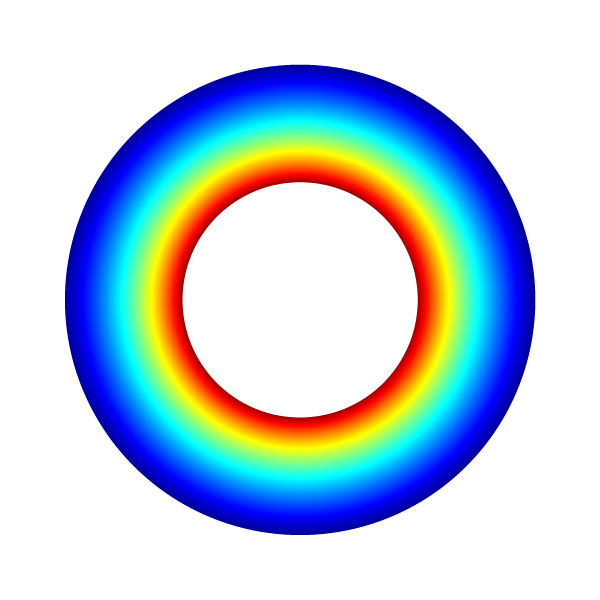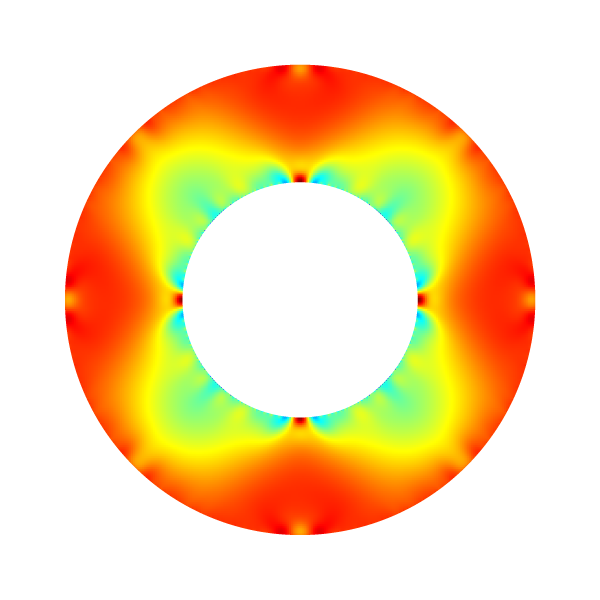# Couette flow between rotating cylinders

We test embedded boundaries by solving the (Stokes) Couette flow between two rotating cylinders.

#include "grid/multigrid.h"
#include "embed.h"
#include "navier-stokes/centered.h"
#include "view.h"

int main()
{
origin (-L0/2., -L0/2.);

stokes = true;
TOLERANCE = 1e-5;
for (N = 16; N <= 256; N *= 2)
run();
}

scalar un[];

#define WIDTH 0.5

event init (t = 0) {

The viscosity is unity.

  mu = fm;

The geometry of the embedded boundary is defined as two cylinders of radii 0.5 and 0.25.

  solid (cs, fs, difference (sq(0.5) - sq(x) - sq(y),
sq(0.25) - sq(x) - sq(y)));

The outer cylinder is fixed and the inner cylinder is rotating with an angular velocity unity.

  u.n[embed] = dirichlet (x*x + y*y > 0.14 ? 0. : - y);
u.t[embed] = dirichlet (x*x + y*y > 0.14 ? 0. :   x);

We initialize the reference velocity field.

  foreach()
un[] = u.y[];
}

We look for a stationary solution.

event logfile (t += 0.01; i <= 1000) {
double du = change (u.y, un);
if (i > 0 && du < 1e-6)
return 1; /* stop */
}

We compute error norms and display the angular velocity, pressure and error fields using bview.

#define powerlaw(r,N) (r*(pow(0.5/r, 2./N) - 1.)/(pow(0.5/0.25, 2./N) - 1.))

event profile (t = end)
{
scalar utheta[], e[];
foreach() {
double theta = atan2(y, x), r = sqrt(x*x + y*y);
if (cs[] > 0.) {
utheta[] = - sin(theta)*u.x[] + cos(theta)*u.y[];
e[] = utheta[] - powerlaw (r, 1.);
}
else
e[] = p[] = utheta[] = nodata;
}

norm n = normf (e);
fprintf (stderr, "%d %.3g %.3g %.3g %d %d %d %d %d\n",
N, n.avg, n.rms, n.max, i, mgp.i, mgp.nrelax, mgu.i, mgu.nrelax);
dump();

draw_vof ("cs", "fs", filled = -1, fc = {1,1,1});
save ("utheta.png");

draw_vof ("cs", "fs", filled = -1, fc = {1,1,1});
save ("p.png");

draw_vof ("cs", "fs", filled = -1, fc = {1,1,1});
save ("e.png");

if (N == 32)
foreach() {
double theta = atan2(y, x), r = sqrt(x*x + y*y);
fprintf (stdout, "%g %g %g %g %g %g %g\n",
r, theta, u.x[], u.y[], p[], utheta[], e[]);
}
}

## ResultsAngular velocityPressure fieldError field

set xlabel 'r'
set ylabel 'u_theta'
powerlaw(r,N)=r*((0.5/r)**(2./N) - 1.)/((0.5/0.25)**(2./N) - 1.)
set grid
set arrow from 0.25, graph 0 to 0.25, graph 1 nohead
set arrow from 0.5, graph 0 to 0.5, graph 1 nohead
plot [0.2:0.55][-0.05:0.35]'out' u 1:6 t 'numerics', powerlaw(x,1.) t 'theory'Velocity profile (N = 32) (script)

Convergence is close to second-order.

unset arrow
set xrange [*:*]
ftitle(a,b) = sprintf("%.3f/x^{%4.2f}", exp(a), -b)
f(x) = a + b*x
fit f(x) 'log' u (log($1)):(log($4)) via a,b
f2(x) = a2 + b2*x
fit f2(x) '' u (log($1)):(log($2)) via a2,b2
set xlabel 'Resolution'
set logscale
set xtics 8,2,1024
set ytics format "% .0e"
set grid ytics
set cbrange [1:2]
set xrange [8:512]
set ylabel 'Error'
set yrange [*:*]
set key top right
plot '' u 1:4 pt 6 t 'max', exp(f(log(x))) t ftitle(a,b), \
'' u 1:2 t 'avg', exp(f2(log(x))) t ftitle(a2,b2)Error convergence (script)